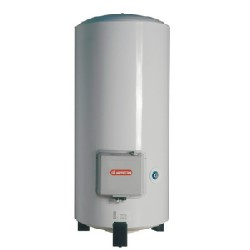# Calculation of hot water heater energy consumption and costsHow much cost your water heating system?
If you want to know the electricity consumption of your hot water tank, and the cost of it, you can use the quick and simple calculator below.

## Calculator of energy and costs for hot water heater

Enter your own values in the white boxes, results are displayed in the green boxes.

### Calculator

V (volume in l/day) : liter/day
Temperature of hot water Th (in °C) : °C
Temperature of cold water Tc (in °C) : °C
Delta T (in °C) : °C
PR :
E (Energy in kWh) : kWh
Currency :
Cost of energy :   /kWh
Total amount of electricity bill per day :
Total amount of electricity bill per year :

## Formula to calculate electricity or gas consumption of water heating

A simple formula to estimate the energy required to heat a volume of water is :
E = C*V*DeltaT/PR
Where E = energy in kWh
C = Specific heat of water - 4.187 kJ/kgK, or 1,163 Wh/kg°C
V = volume of water to heat
deltaT = Th-Tc
Th = temperature of hot water
Tc = temperature of input cold water
PR = performance ratio (it includes losses of heat through pipes and tank), default value = 0.9